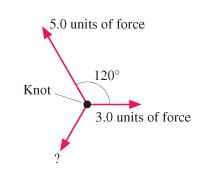# Problem: The figure shows three ropes tied together in a knot. One of your friends pulls on a rope with 3.0 units of force and another pulls on a second rope with 5.0 units of force. (A) How hard must you pull on the third rope to keep the knot from moving? (B) In what direction must you pull on the third rope to keep the knot from moving?

###### FREE Expert Solution

Newton's second law:

$\overline{){\mathbf{\Sigma }}{\mathbf{F}}{\mathbf{=}}{\mathbf{m}}{\mathbf{a}}}$

Resolving 2D vector components:

(A)

Consider a standard coordinate system. Positive x points to the right while positive y points upward.

The components of the unknown force are Fx and Fy.

80% (93 ratings)###### Problem Details

The figure shows three ropes tied together in a knot. One of your friends pulls on a rope with 3.0 units of force and another pulls on a second rope with 5.0 units of force. (A) How hard must you pull on the third rope to keep the knot from moving? (B) In what direction must you pull on the third rope to keep the knot from moving?